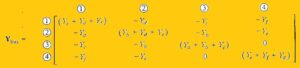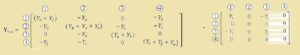Hello, readers welcome to new post. In this post, we will learn how to solve node equations. Node equation re technique that used to solve different circuits in the electrical power system in a simple way. It makes our work easy and simple related to find the parameters used in the system like volts current and power.

So let get started

### How to Solve Node Equation

• The common point in the circuitry where two elements of circuitry ar linked is called node.
• Normally KCL and KVL is used to solve the different equation existing in the circuitry.
• To the understanding of node, equation let’s take a circuitry and solve it.• In this circuitry, node are denoted with a number in the circle.
• Current sources are linked at nodes number three and four and other elements are taken as admittance.
• The is the usage of single subscript notation to make the voltage values of every node according to the reference node
• If we apply KCL at node number one current is moving away from the node so equation will be

(V1-V3)Yc+(V1-V2)Yd+(V1-V4) Vf=0

• At not number three we have

V3Ya-(V3-V2)Yb+(V3-V1)Yc=I3

• After simplifying this expression we have
• For node number one

V1(Yc+Yd+Yf)-V2Yd-V3Yc-V4Yf=0

• For node three

-V1Yc-V2Yb+V3(Ya+Yb+YC)=I3

• All the diagonal component Yii is equal to the addition of admittance linked to the node.
• The component that are not in diagonal equal to the negative of total admittance linked among the node i and j